## Harmonic Number

A number of the form(1)

This can be expressed analytically as(2)

whereis the Euler-Mascheroni Constant and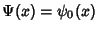is the Digamma Function. The number formed by taking alternate signs in the sum also has an analytic solution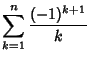(3)(4)

The first few harmonic numbersare 1,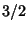,,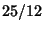,, ... (Sloane's A001008 and A002805). The Harmonic Numberis never an Integer (except for), which can be proved by using the strong triangle inequality to show that the 2-adic value ofis greater than 1 for. The harmonic numbers have Odd Numerators and Even Denominators. Theth harmonic number is given asymptotically by(5)

whereis the Euler-Mascheroni Constant (Conway and Guy 1996). Gosper gave the interesting identity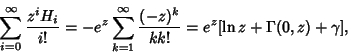(6)

where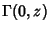is the incomplete Gamma Function andis the Euler-Mascheroni Constant. Borwein and Borwein (1995) show that(7)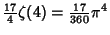(8)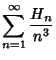(9)

whereis the Riemann Zeta Function. The first of these had been previously derived by de Doelder (1991), and the last by Euler (1775). These identities are corollaries of the identity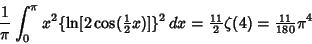(10)

(Borwein and Borwein 1995). Additional identities due to Euler are(11)(12)

for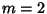, 3, ... (Borwein and Borwein 1995), whereis Apéry's Constant. These sums are related to so-called Euler Sums.

Conway and Guy (1996) define the second harmonic number by(13)

the third harmonic number by(14)

and theth harmonic number by(15)

A slightly different definition of a two-index harmonic number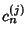is given by Roman (1992) in connection with the Harmonic Logarithm. Roman (1992) defines this by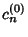(16)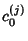(17)

plus the recurrence relation(18)

For general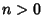and, this is equivalent to(19)

and for, it simplifies to(20)

For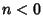, the harmonic number can be written(21)

whereis the Roman Factorial andis a Stirling Number of the First Kind.

A separate type of number sometimes also called a harmonic number'' is a Harmonic Divisor Number (or Ore Number).

See also Apéry's Constant, Euler Sum, Harmonic Logarithm, Harmonic Series, Ore Number

References

Borwein, D. and Borwein, J. M. On an Intriguing Integral and Some Series Related to.'' Proc. Amer. Math. Soc. 123, 1191-1198, 1995.

Conway, J. H. and Guy, R. K. The Book of Numbers. New York: Springer-Verlag, pp. 143 and 258-259, 1996.

de Doelder, P. J. On Some Series Containing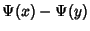andfor Certain Values ofand.'' J. Comp. Appl. Math. 37, 125-141, 1991.

Roman, S. The Logarithmic Binomial Formula.'' Amer. Math. Monthly 99, 641-648, 1992.

Sloane, N. J. A. Sequences A001008/M2885 and A002805/M1589 in An On-Line Version of the Encyclopedia of Integer Sequences.'' http://www.research.att.com/~njas/sequences/eisonline.html and Sloane, N. J. A. and Plouffe, S. The Encyclopedia of Integer Sequences. San Diego: Academic Press, 1995.